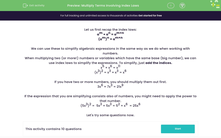# Multiply Terms Involving Index Laws

In this worksheet, students will multiply terms using index laws to simplify expressions.Key stage:  KS 4

Year:  GCSE

GCSE Subjects:   Maths

GCSE Boards:   OCR

Curriculum topic:   Indices and Surds

Curriculum subtopic:   Standard Form

Difficulty level:#### Worksheet Overview

Let us first recap the index laws:

am × an = am+n

(am)n = am×n

We can use these to simplify algebraic expressions in the same way as we do when working with numbers.

When multiplying two (or more!) numbers or variables which have the same base (big number), we can use index laws to simplify the expressions. To simplify, just add the indices.

x× x6 = x11

(x3)2 = x3 × x3 = x6

If you have two or more numbers, you should multiply them out first.

3x6 × 7x3 = 21x9

If the expression that you are simplifying consists also of numbers, you might need to apply the power to that number.

(5x3)2 =  5x3 × 5x3 = 5× x6  = 25x6

Let's try some questions now.

### What is EdPlace?

We're your National Curriculum aligned online education content provider helping each child succeed in English, maths and science from year 1 to GCSE. With an EdPlace account you’ll be able to track and measure progress, helping each child achieve their best. We build confidence and attainment by personalising each child’s learning at a level that suits them.

Get started